# ThinkPHP容器源码深度解析(5)

+关注继续查看

#### 实战案例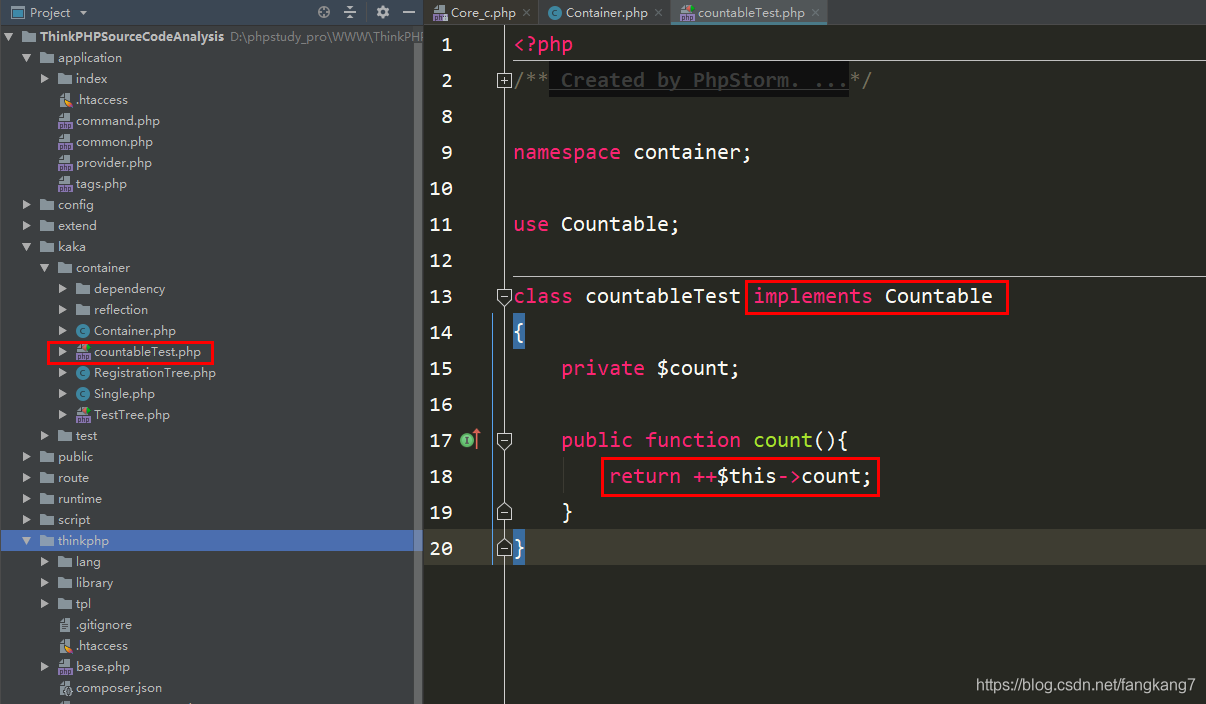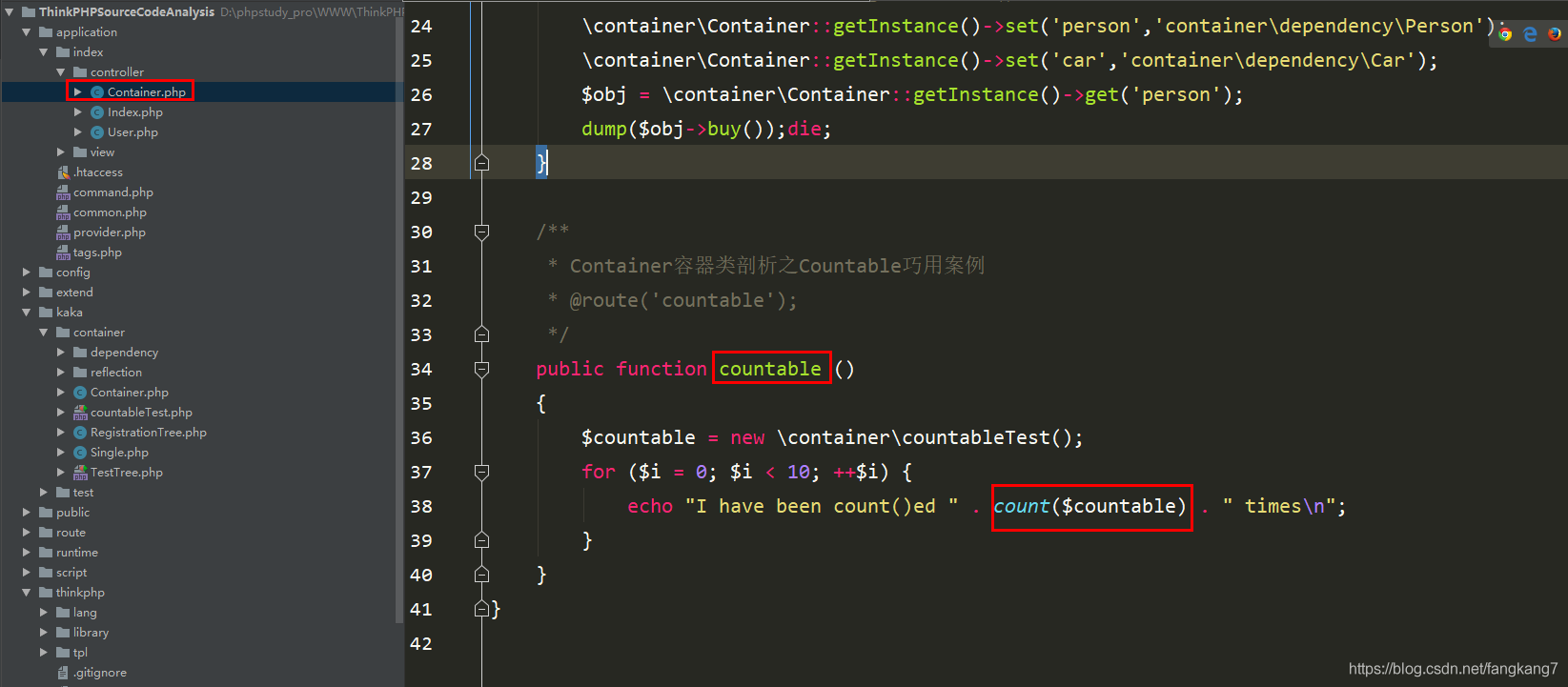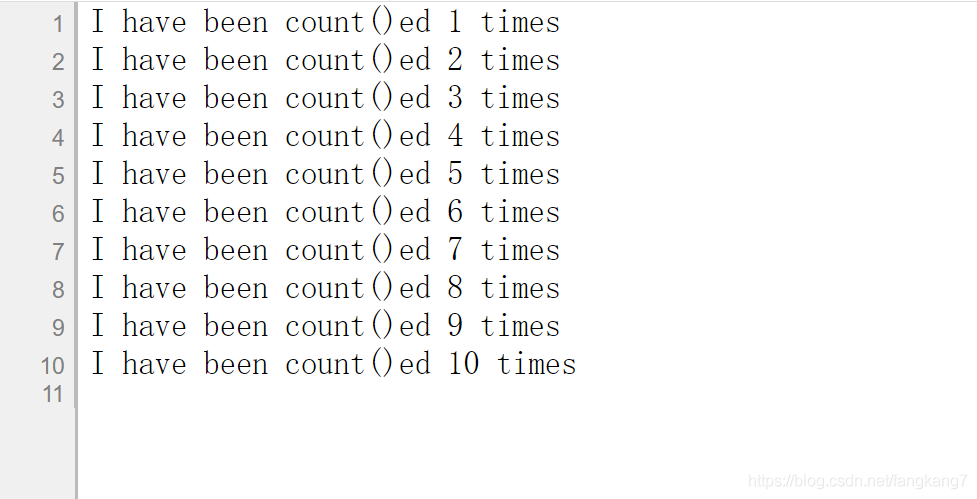Countable中的count()跟平时使用count()方法有什么区别

int count ( mixed $array_or_countable [, int$mode = COUNT_NORMAL ] )

#### count你不知道的用法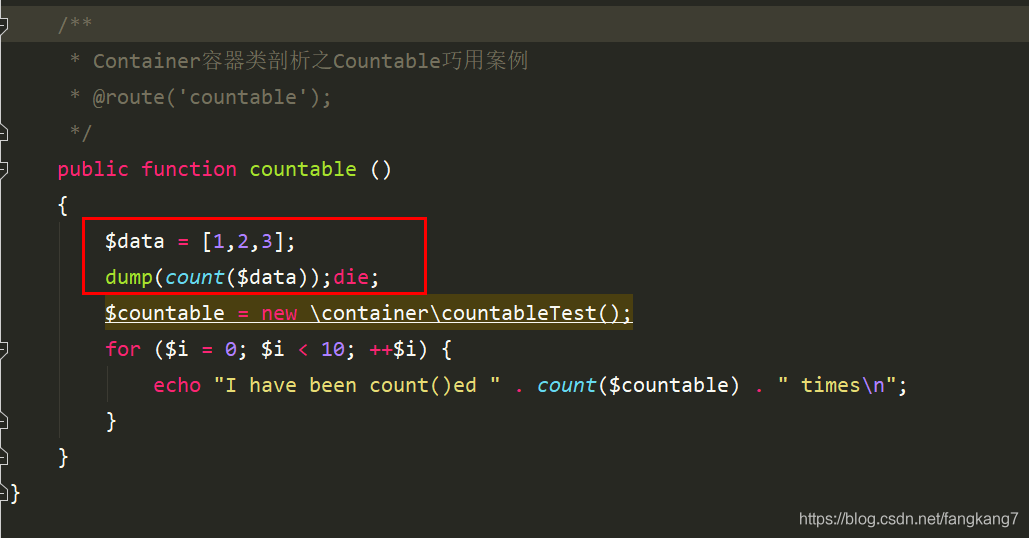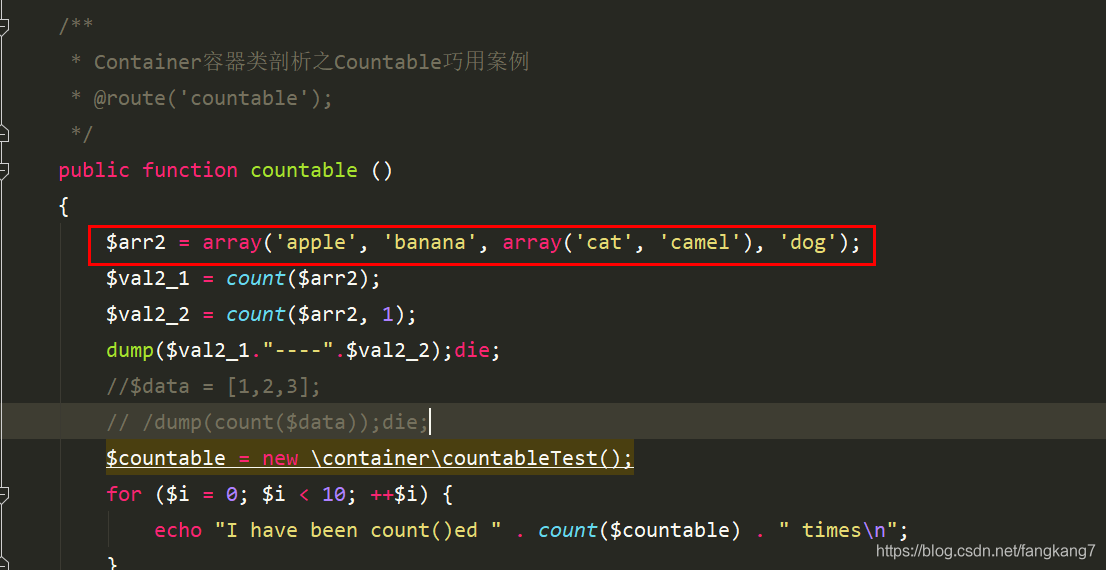## 七、Container容器类剖析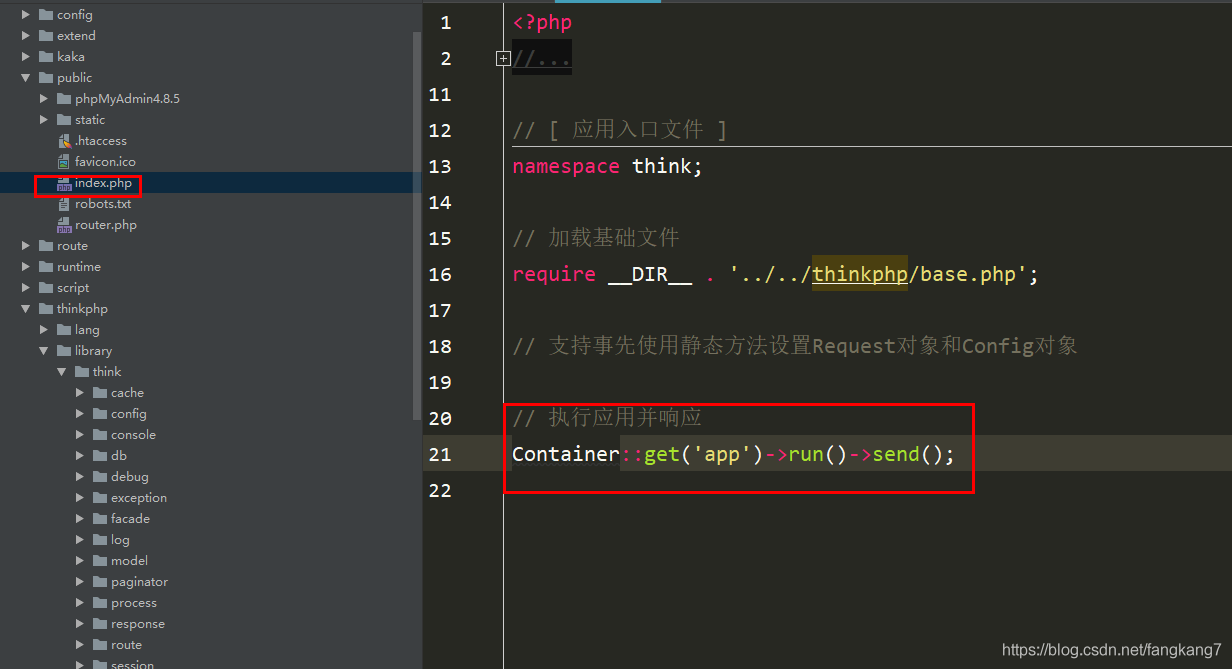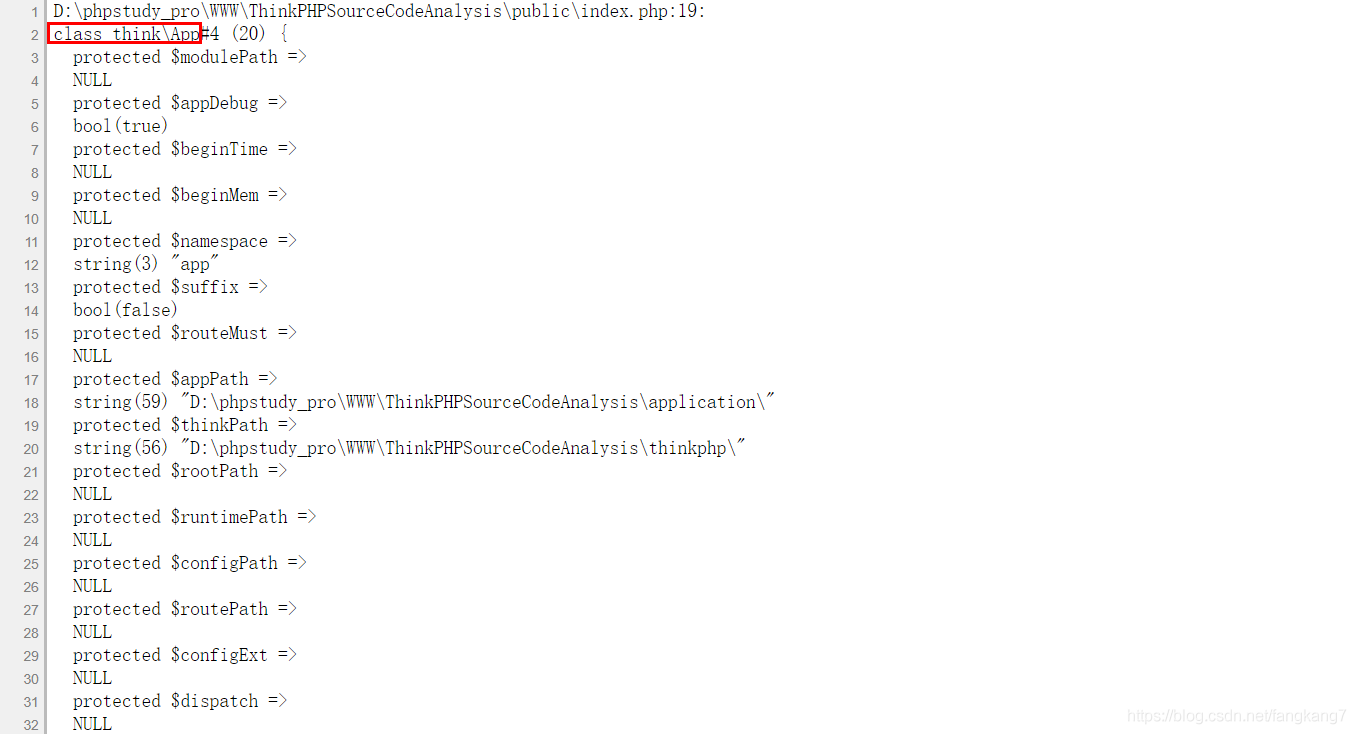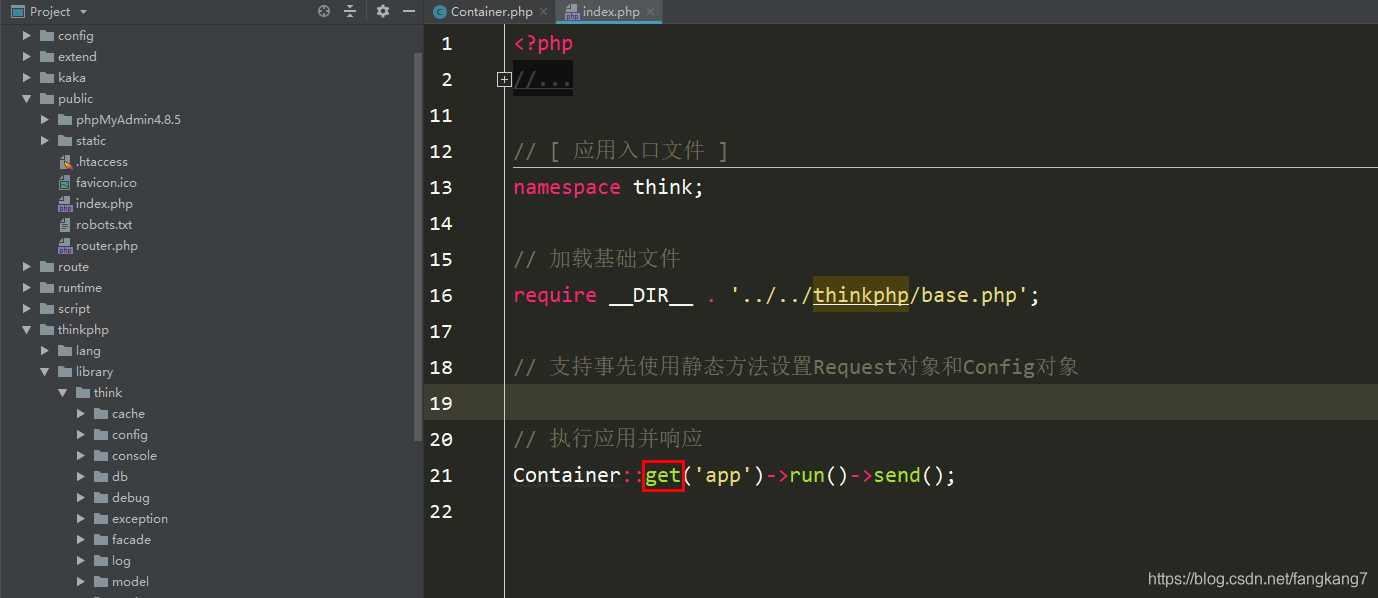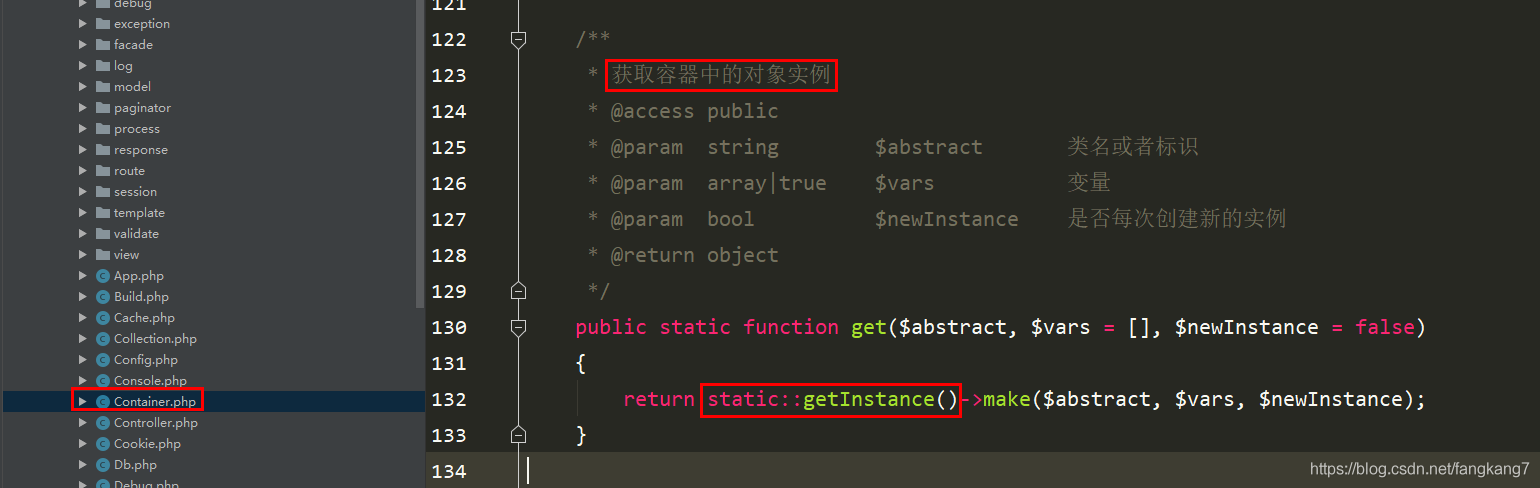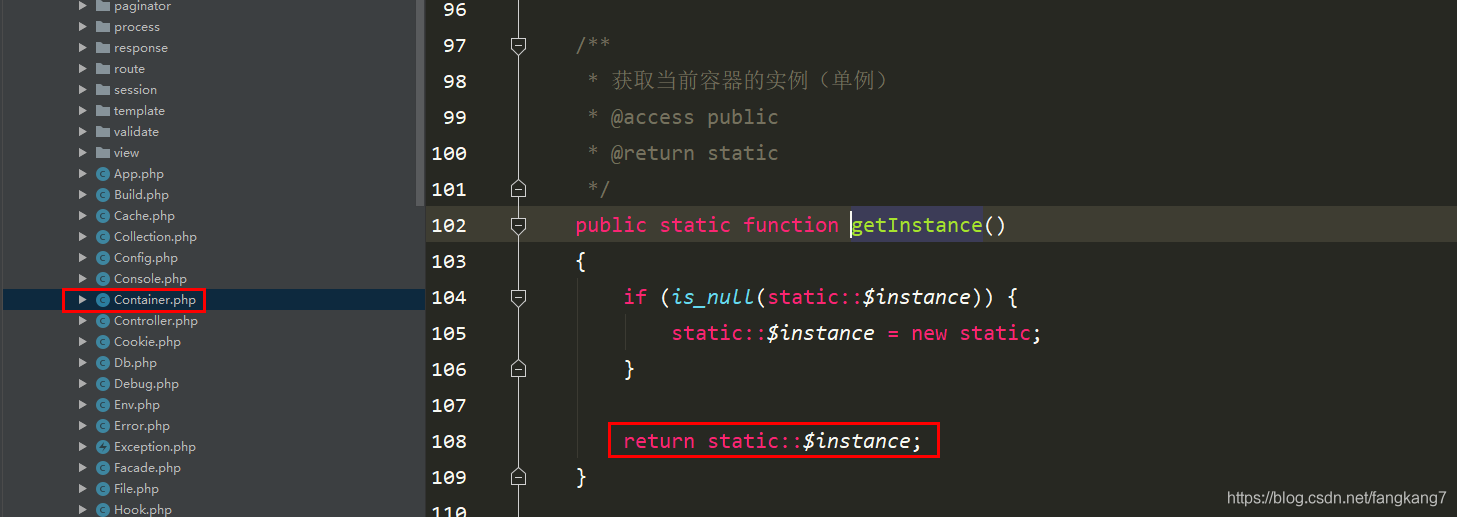#### Container实例调用make方法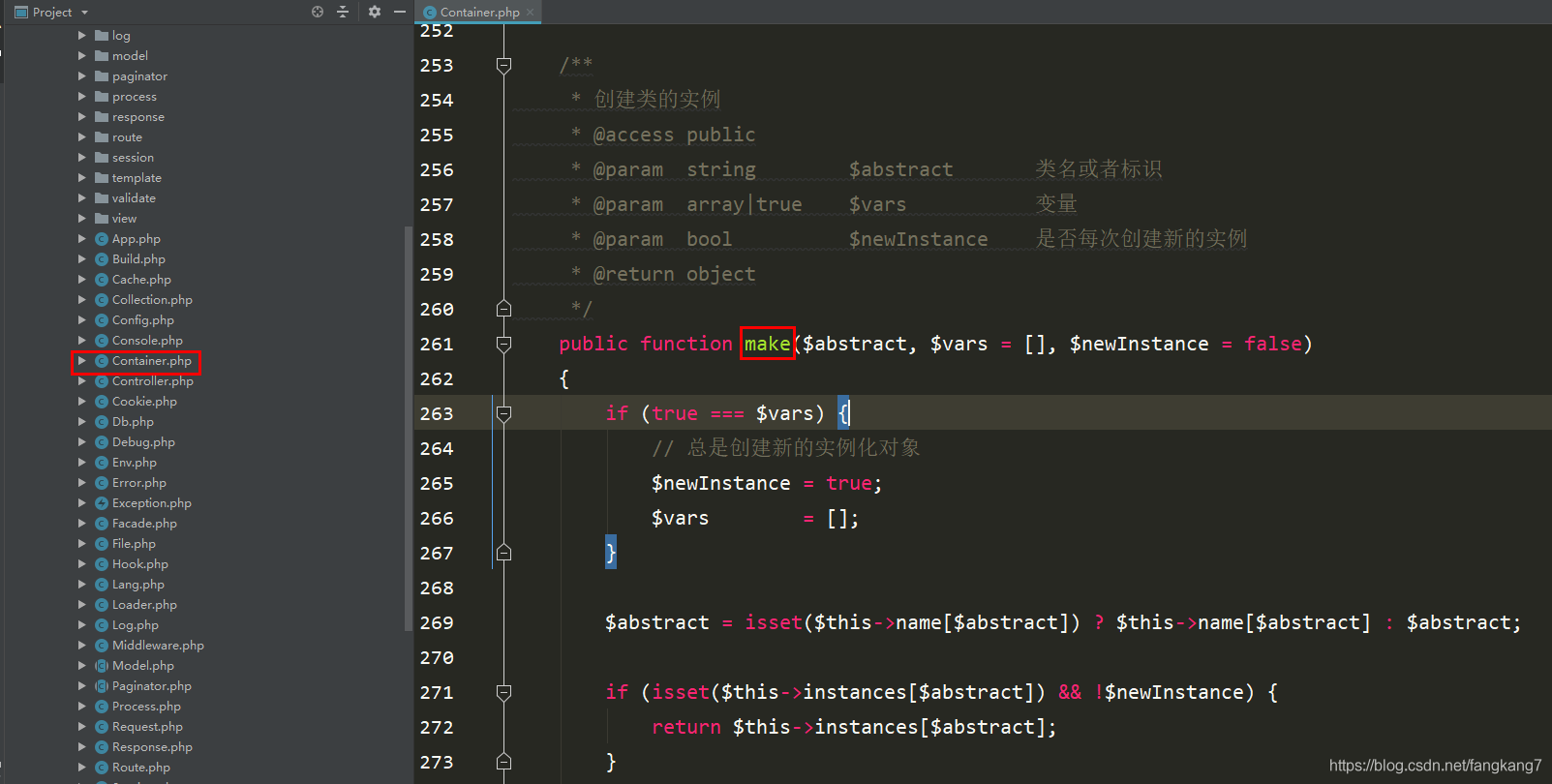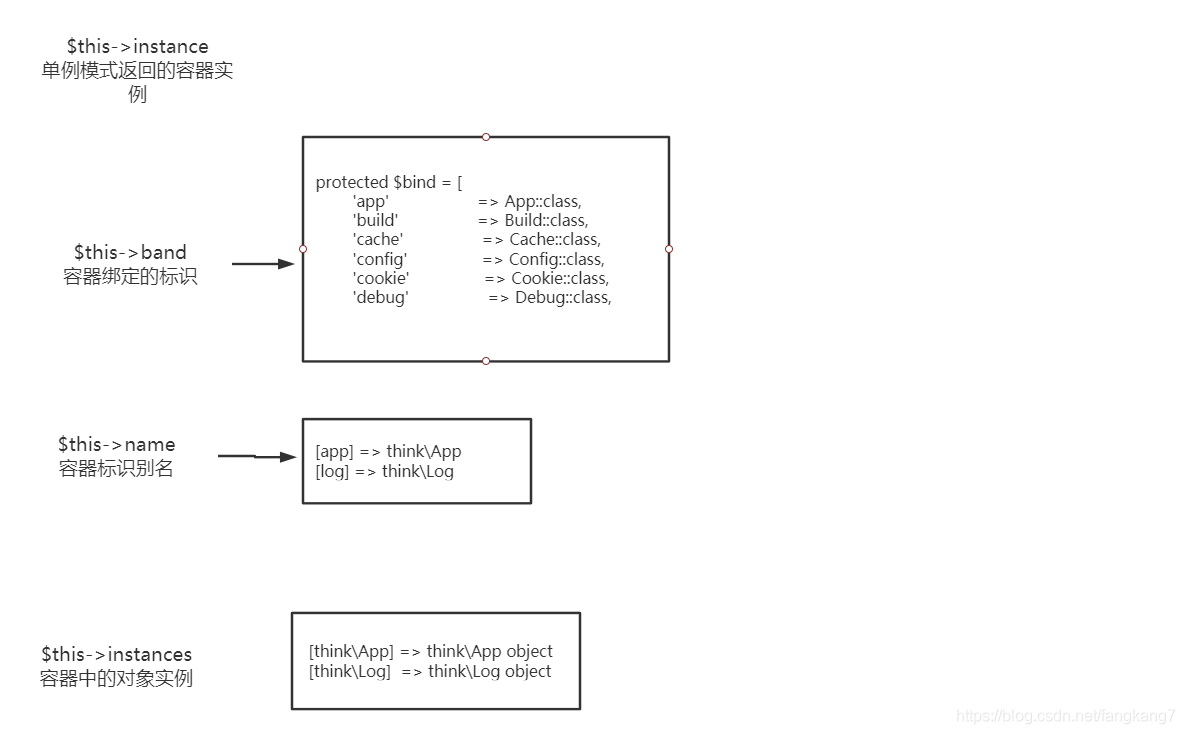/**
* 创建类的实例
* @access public
* @param  string        $abstract 类名或者标识 * @param array|true$vars           变量
* @param  bool          $newInstance 是否每次创建新的实例 * @return object */ public function make($abstract, $vars = [],$newInstance = false)
{
// 判断$vars这个变量是否为true if (true ===$vars) {
// 总是创建新的实例化对象
$newInstance = true;$vars        = [];
}

// app  这里就是在容器别名里获取传递过来的app    如果没有则就是app
$abstract = isset($this->name[$abstract]) ?$this->name[$abstract] :$abstract;

// 从容器实例中获取  如果存在则直接返回对应的实例  也就是使用注册树模式
if (isset($this->instances[$abstract]) && !$newInstance) { return$this->instances[$abstract]; } // think\App 从容器标识中获取 if (isset($this->bind[$abstract])) { // 将think\App 复制给$concrete变量
$concrete =$this->bind[$abstract]; // 用于代表匿名函数的类 判断是不是闭包 if ($concrete instanceof Closure) {
$object =$this->invokeFunction($concrete,$vars);
} else {
// $this->name['app'] = think\App$this->name[$abstract] =$concrete;
// 在执行一次本类的make方法，也就是本方法
return $this->make($concrete, $vars,$newInstance);
}
} else {
$object =$this->invokeClass($abstract,$vars);
}

if (!$newInstance) {$this->instances[$abstract] =$object;
}

return $object; }  这是第二次执行流程  public function make($abstract, $vars = [],$newInstance = false)
{
// 判断$vars这个变量是否为true if (true ===$vars) {
// 总是创建新的实例化对象
$newInstance = true;$vars        = [];
}

// app  这里就是在容器别名里获取传递过来的app    如果没有则就是app
// 第二次执行时 $abstract = think\App$abstract = isset($this->name[$abstract]) ? $this->name[$abstract] : $abstract; // 从容器实例中获取 如果存在则直接返回对应的实例 也就是使用注册树模式 if (isset($this->instances[$abstract]) && !$newInstance) {
return $this->instances[$abstract];
}

// think\App 从容器标识中获取
// 第二次执行$this->bind['think\App']不存在走else if (isset($this->bind[$abstract])) { // 将think\App 复制给$concrete变量
$concrete =$this->bind[$abstract]; // 用于代表匿名函数的类 判断是不是闭包 if ($concrete instanceof Closure) {
$object =$this->invokeFunction($concrete,$vars);
} else {
// $this->name['app'] = think\App$this->name[$abstract] =$concrete;
// 在执行一次本类的make方法，也就是本方法
// think\App
return $this->make($concrete, $vars,$newInstance);
}
} else {
// think\App
$object =$this->invokeClass($abstract,$vars);
}

if (!$newInstance) { // 把创建的容器存起来 //$this->instances['think\App'] = $object;$this->instances[$abstract] =$object;
}

return $object; }  public function invokeClass($class, $vars = []) { try { /** * ReflectionClass Object ( [name] => think\App ) */ // 这里就是之前文章提到的反射$reflect = new ReflectionClass($class); if ($reflect->hasMethod('__make')) {
$method = new ReflectionMethod($class, '__make');

if ($method->isPublic() &&$method->isStatic()) {
$args =$this->bindParams($method,$vars);
return $method->invokeArgs(null,$args);
}
}
// 通过反射获取think\App的构造函数
$constructor =$reflect->getConstructor();

$args =$constructor ? $this->bindParams($constructor, $vars) : []; // 从给出的参数创建一个新的类实例 return$reflect->newInstanceArgs($args); } catch (ReflectionException$e) {
throw new ClassNotFoundException('class not exists: ' . $class,$class);
}
}63980 015959 0Xshell使用SSH远程登录阿里云ECS服务器CentOS7
22339 023581 0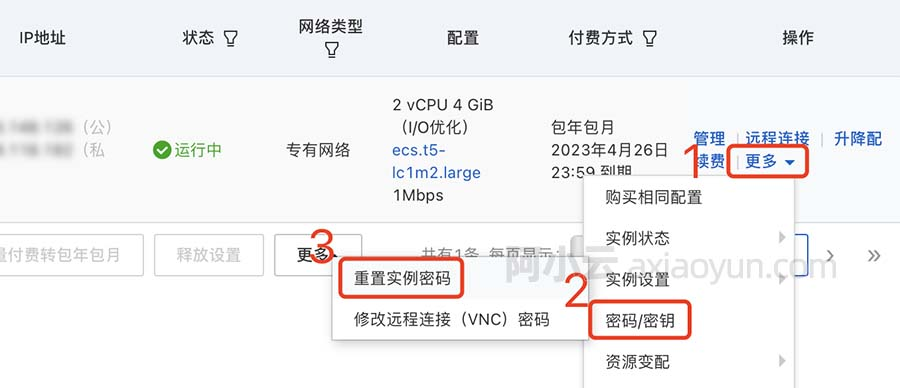22359 019808 0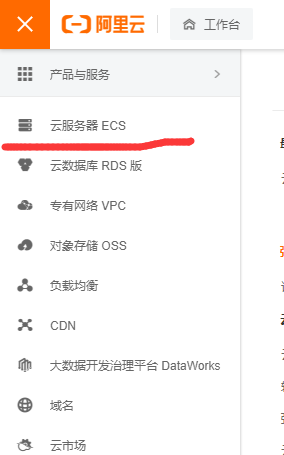14761 036450 0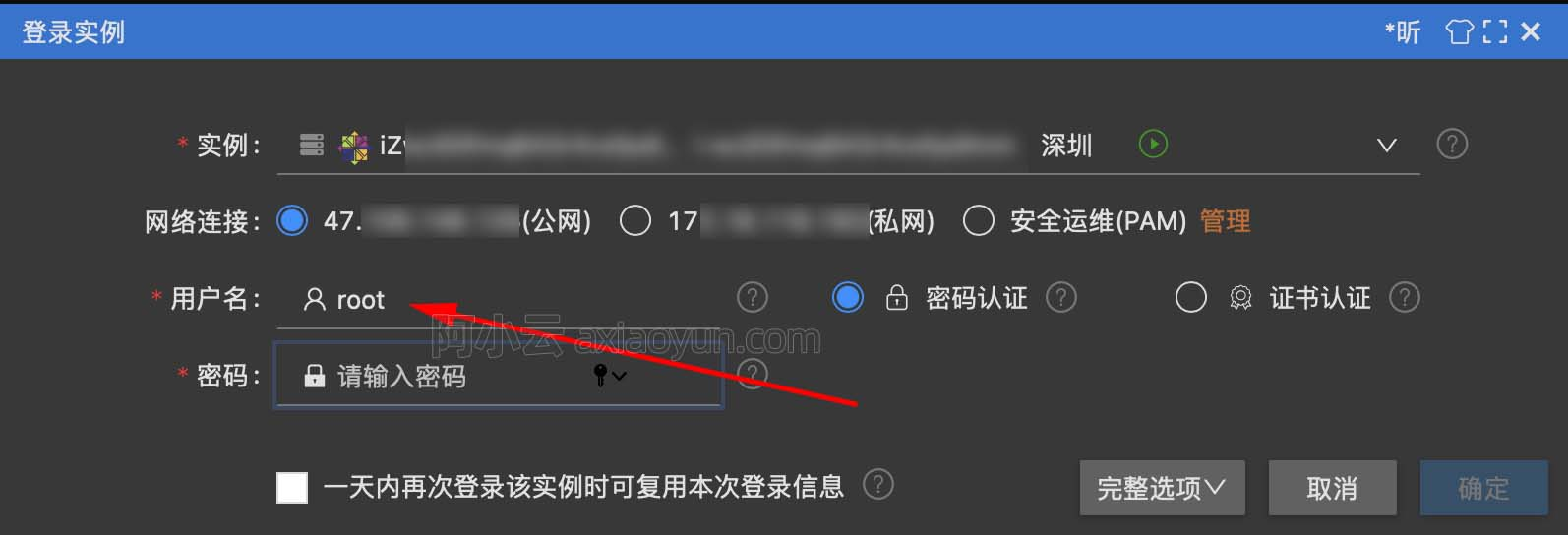16454 014900 0
1039

0

JS零基础入门教程（上册）